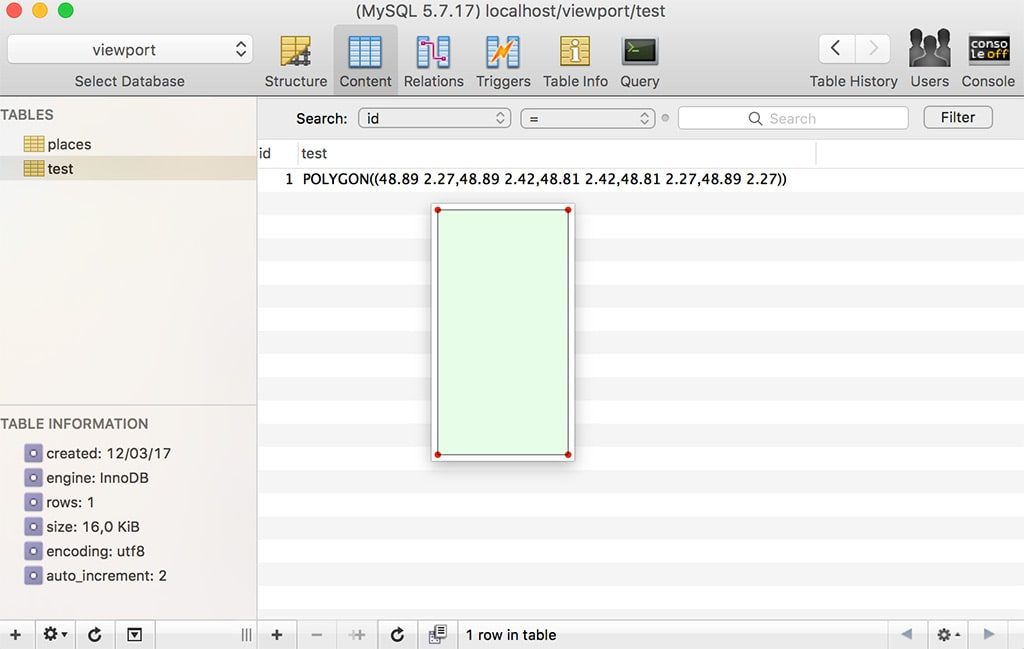# How To Find Points Contained in a Polygon Using MySQL

13 March 2017

MySQL was a bit late to the geospatial party compared to other databases like Postgres, but with the 5.6 and then the 5.7 releases a lot of new GIS features were finally implemented. For instance in 5.7 we got, among other:

• `ST_Distance_Sphere` to compute the distance between two points on a sphere.
• Spatial indexes for InnoDB
• GeoJSON integration which is, quoting from the RFC, “a geospatial data interchange format based on JSON.”

There’s a lot of interesting things we can now accomplish using these new fonctionnalities. Today I’ll showcase a very simple example on how to do a search using a Polygonand a Point. The goal here will be to answer the question “is this point in this city?”.

## Defining Points

First let’s create a “places” table with a name and a set of coordinates. However, instead of storing a latitude and a longitude as two different floats I’ll use a `Point`.

Then let’s add a couple of places in Paris and one in New York for very basic testing. The way to store a new `Point` is very straighforward:

## Filtering

Let’s then try to answer the question “what places in my database are in Paris?”.

### Filtering With `ST_Distance_Sphere`

First let’s try to use MySQL’s `ST_Distance_Sphere` to find the distance between two points. Let’s use the Louvre’s coordinates (48.861105, 2.335337) which is in the center of Paris. We can easily compute the distance using `ST_Distance_Sphere`.

This gives us:

``````Eiffel Tower    4629.968479838098
Pere Lachaise   6598.960247905806
Brooklyn    8500637.22140377
``````

The distance returned is in metters, so everything checks out. If we want to see all points that are less than 10km from the Louvre, we can do:

This is a pretty good approximation to being in Paris, but that’s not perfect. What if I wanted to know if a point was really in Paris and not just close to the center of Paris?

### Filtering With a Polygon and `ST_CONTAINS`

First let’s define a very basic shape enclosing Paris. The coordinates I’ll be using here are a very rough approximation and I only define a rectangle, but that’ll work for this example:

If you look closer, you’ll notice that the last point is the same as the first one and we have 5 points. This is because we need to “close” the polygon.

To double check the result, we can use Sequel Pro’s preview feature and confirm how it looks:Then we can use `ST_CONTAINS` to ask all the places contained in the `@paris` polygon:

Of course I could have skipped the step where I defined `@paris` and called `POLYGON` directly in the query.

## Performances

Geospacial functions are fun to play with and since we can now use spatial indexes in InnoDB, they become actually usable in production. I won’t get into performances in this article, but I recommend reading the Percona article “New GIS Features in MySQL 5.7”.